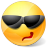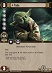# Math Is Fun Forum

Discussion about math, puzzles, games and fun.   Useful symbols: ÷ × ½ √ ∞ ≠ ≤ ≥ ≈ ⇒ ± ∈ Δ θ ∴ ∑ ∫  π  -¹ ² ³ °

You are not logged in.

## #1 2010-04-08 12:43:06

careless25
Real MemberRegistered: 2008-07-24
Posts: 560

### Vectors and Planes

I have a math mid-term tomorrow and I cant do these from the Review. Can you solve/explain them to me ASAP?

1. Determine the components of a vector of length 44 that lies on the line of intersection of the planes with equations 3x - 4y + 9z = 0 and 2y - 9z = 0.

2. The line through a point P(a,0,a) with direction vector (-1,2,-1) intersects the plane 3x + 5y + 2z = 0 at point Q. The line through P with direction vector (-3,2,-1) intersects the plane at point R. For what choice of a is the distance between Q and R equal to 3?

3. Consider the two lines:
L1: (x,y,z) = (2,0,0) + t(1,2,-1)
L2: (x,y,z) = (3,2,3) + s(a,b,1)
where s and t are real numbers. Find a relationship between a and b (independent of s and t) that ensures that L1 and L2 intersect.

For this one, I get the right answer but I m not sure if my method is correct.
I take the 2 points given and subtract them and find the direction vector (1,2,3).
I also take the 2 direction vectors given and subtract them to obtain (a-1,b-2,2).
I make x1=x2, y1=y2, z1=z2.
I get:
a-1 = 1
a = 2

b-2 = 2
b = 4

3 = 2??

and a = 1/2 b is the answer.

4. In the following system of equations, k is a real number.
-2x + 4y + z = k + 1
kx + z = 0
y + kz = 0

a. For what valuess of k does the system:
i) have no solutions?
ii) have exactly one solution?
iii) have an infinite amount of solutions?

C25

Offline

## #2 2018-05-11 15:59:26

Monox D. I-Fly
MemberFrom: Indonesia
Registered: 2015-12-02
Posts: 2,000

### Re: Vectors and Planes

Any idea how to answer number 4? I tried kx + z = y + kz and got this:
kx – y + z – kz = 0
kx – y + (1 – k)z = 0
To make the system have no solution or infinite amount of solutions, I think kx – y + (1 – k)z is a multiple of -2x + 4y + z thus I multiplied it by -4 and got -4kx + 4y + (-4 + 4k)z = 0. From the x coefficient, I got k = 1/2, but from the z coefficient I got 5/4. Different answers. Was what I do wrong? What is the proper way to solve it? Thanks.

Actually I never watch Star Wars and not interested in it anyway, but I choose a Yoda card as my avatar in honor of our great friend bobbym who has passed away.
May his adventurous soul rest in peace at heaven.

Offline

## #3 2018-05-11 20:09:04

BobRegistered: 2010-06-20
Posts: 9,299

### Re: Vectors and Planes

hi Monox D. I-Fly

I thought 'who is this examiner to set the exam for a Saturday'? but then I noticed the post date.Let's hope he did well.

This is what I did for number 4:

Note: It's easy to eliminate x and y to get an equation in z.

2 .... gives x = -z/k          and      3 ... gives y = -kz so substitute these into 1 to get an equation for z.

It has a quadratic denominator so the roots will lead to impossible to solve.

I used the function plotter https://www.mathsisfun.com/data/function-grapher.php? to see what values of z are possible.  There are three asymptotes, two vertical at the roots mentioned above and one horizontal.

As this is a valid function, any value of k that leads to a (unique) value of z means a single set of values for z, x and y.  That's most values of k.  There are two values at approximately - 0.6 and +0.8 (if my algebra is correct) where z tends to infinity so no solutions.  I cannot find any values of k where more than one solution exists.  At one point in my algebra I multiplied through by k to remove the fraction so I separately tested k=0 and that leads to a single solution too.

Bob

Children are not defined by school ...........The Fonz
You cannot teach a man anything;  you can only help him find it within himself..........Galileo Galilei
Sometimes I deliberately make mistakes, just to test you!  …………….BobOffline